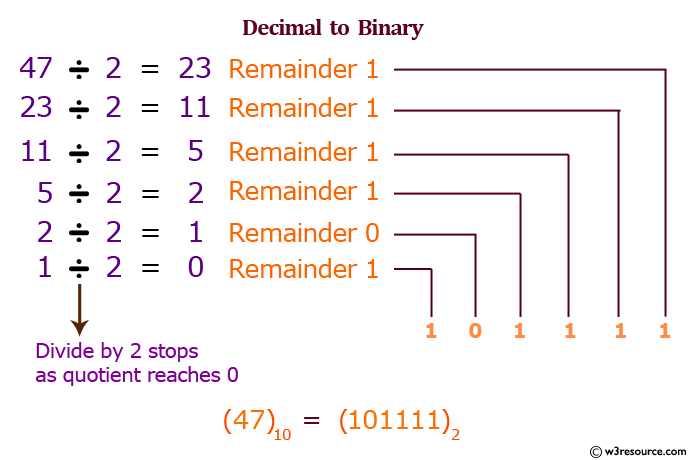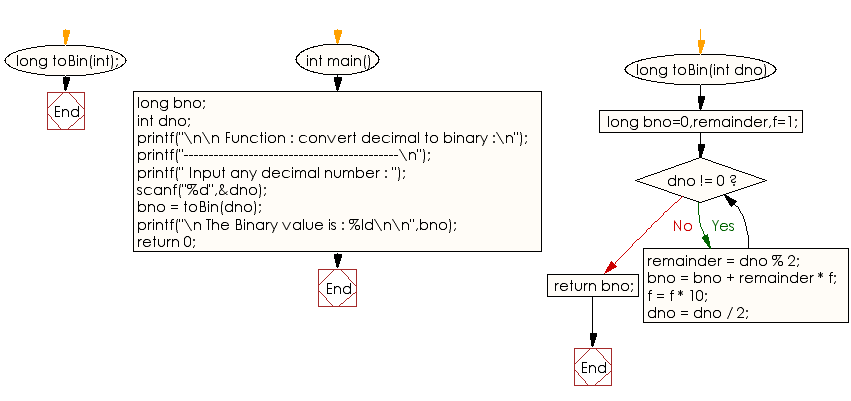﻿ C Program: Convert decimal to binary - w3resource

# C Exercises: Convert decimal to binary

## C Function : Exercise-6 with Solution

Write a program in C to convert a decimal number to a binary number using the function.

Pictorial Presentation:Sample Solution:

C Code:

``````#include<stdio.h>

long toBin(int);

int main()
{
long bno;
int dno;
printf("\n\n Function : convert decimal to binary :\n");
printf("-------------------------------------------\n");
printf(" Input any decimal number : ");
scanf("%d",&dno);
bno = toBin(dno);
printf("\n The Binary value is : %ld\n\n",bno);

return 0;
}
long toBin(int dno)
{
long bno=0,remainder,f=1;
while(dno != 0)
{
remainder = dno % 2;
bno = bno + remainder * f;
f = f * 10;
dno = dno / 2;
}
return bno;
}
```
```

Sample Output:

``` Function : convert decimal to binary :
-------------------------------------------
Input any decimal number : 65

The Binary value is : 1000001
```

Explanation:

```long toBin(int dno) {
long bno = 0, remainder, f = 1;
while (dno != 0) {
remainder = dno % 2;
bno = bno + remainder * f;
f = f * 10;
dno = dno / 2;
}
return bno;
}
```

The function 'toBin' takes a single argument 'dno' of type int. It converts the input number 'dno' from decimal to binary by repeatedly dividing 'dno' by 2 and storing the remainders in reverse order. The function initializes three local variables, 'bno', 'remainder', and 'f', to 0, 1, and 1 respectively. It then enters a loop that continues as long as 'dno' is not equal to 0. In each iteration of the loop, the remainder of 'dno' divided by 2 is computed and stored in remainder. The value of 'bno' is updated by adding remainder multiplied by 'f'. The value of f is updated by multiplying it with 10. Finally, 'dno' is updated by dividing it by 2. This loop computes the binary equivalent of 'dno' and returns it as a long integer.

Time complexity and space complexity:

The time complexity of this function is O(log n), where n is the input number 'dno', because the loop iterates log_2(n) times to compute the binary equivalent of dno.

The space complexity of this function is O(1), as it only uses a fixed amount of memory to store the three integer variables bno, remainder, and f.

Flowchart:C Programming Code Editor:

What is the difficulty level of this exercise?

Test your Programming skills with w3resource's quiz.

﻿